Astrophysics

Physicists count four fundamental forces between matter particles, but none impacts the large-scale structure of the universe more than gravity. Unlike the other forces, gravity is felt by every body, large or small; it links every last atom in the universe.

Gravity was also the first force to be understood through a modern scientific model. Inaugural scientists like Galileo and Newton pieced together a theory of gravity in the $1600$'s that is still used in applications today. The techniques of modern astronomy arose from their desire to understand and predict the motions of planets under gravity's action.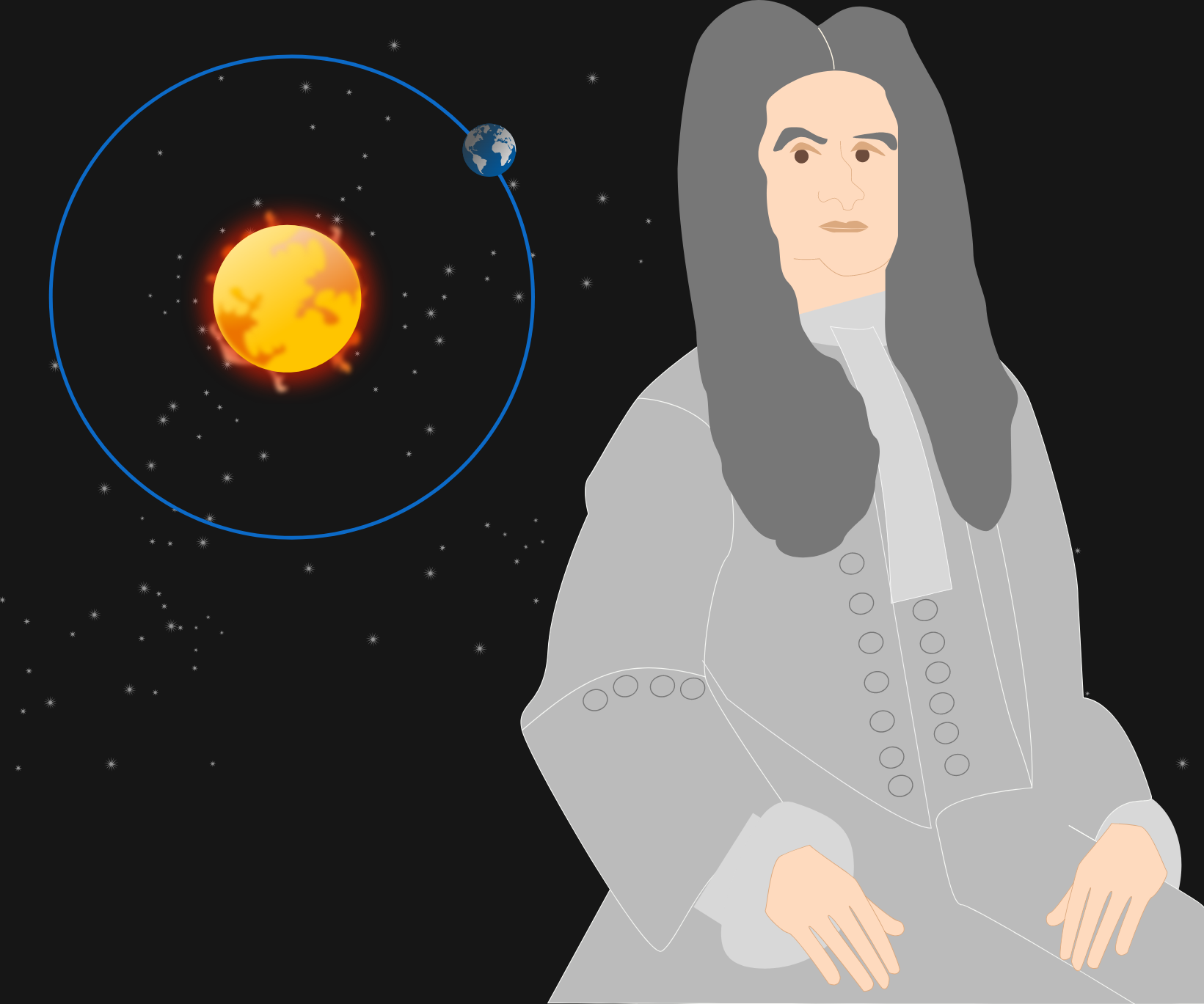To get a feel for how gravity impacts the structure of the visible universe, let's start by calculating and comparing some typical gravitational forces in our solar system.

Gravity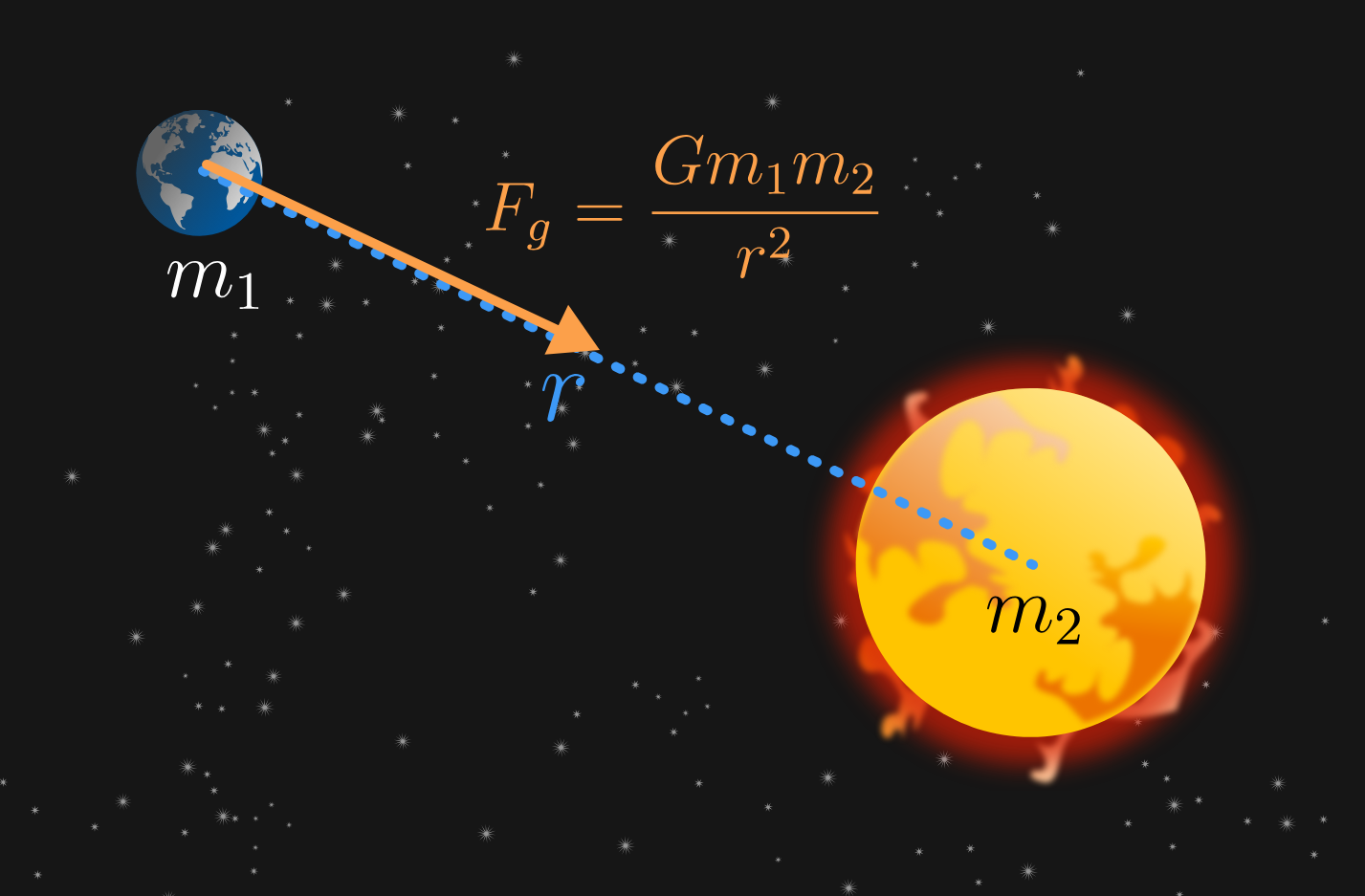The force of gravity can be calculated from a simple quantitative law: gravity between two masses $m_1$ and $m_2$ separated by a distance $r$ is $F_g=\frac{Gm_1 m_2}{r^2},$ where $G=\SI[per-mode=symbol]{6.67e-11}{\meter\cubed\per\kilo\gram\per\second\squared}.$ The distance $r$ is measured from the center of one object to the center of the other.

How large is the gravitational force of the Sun on Earth? Apply the gravitational force law above to the Sun-Earth system.


Details and Assumptions:

• Earth's mass is $\SI{5.97e24}{\kilo\gram}.$
• The Sun's mass is $\SI{1.99e30}{\kilo\gram}.$
• In the next chapter, we will see how the ancient Greeks measured the Earth-Sun distance: $d_\text{Earth}=\SI{1.5e11}{\meter}.$

Gravity

The Sun's gravity is responsible for shepherding the planets around nearly circular orbits in our solar system. The mass of Saturn, the ringed gas giant in our solar system, is $95.16$ times Earth's. In orbit, Saturn's distance from the Sun is $9.57$ times the distance from Earth to the Sun.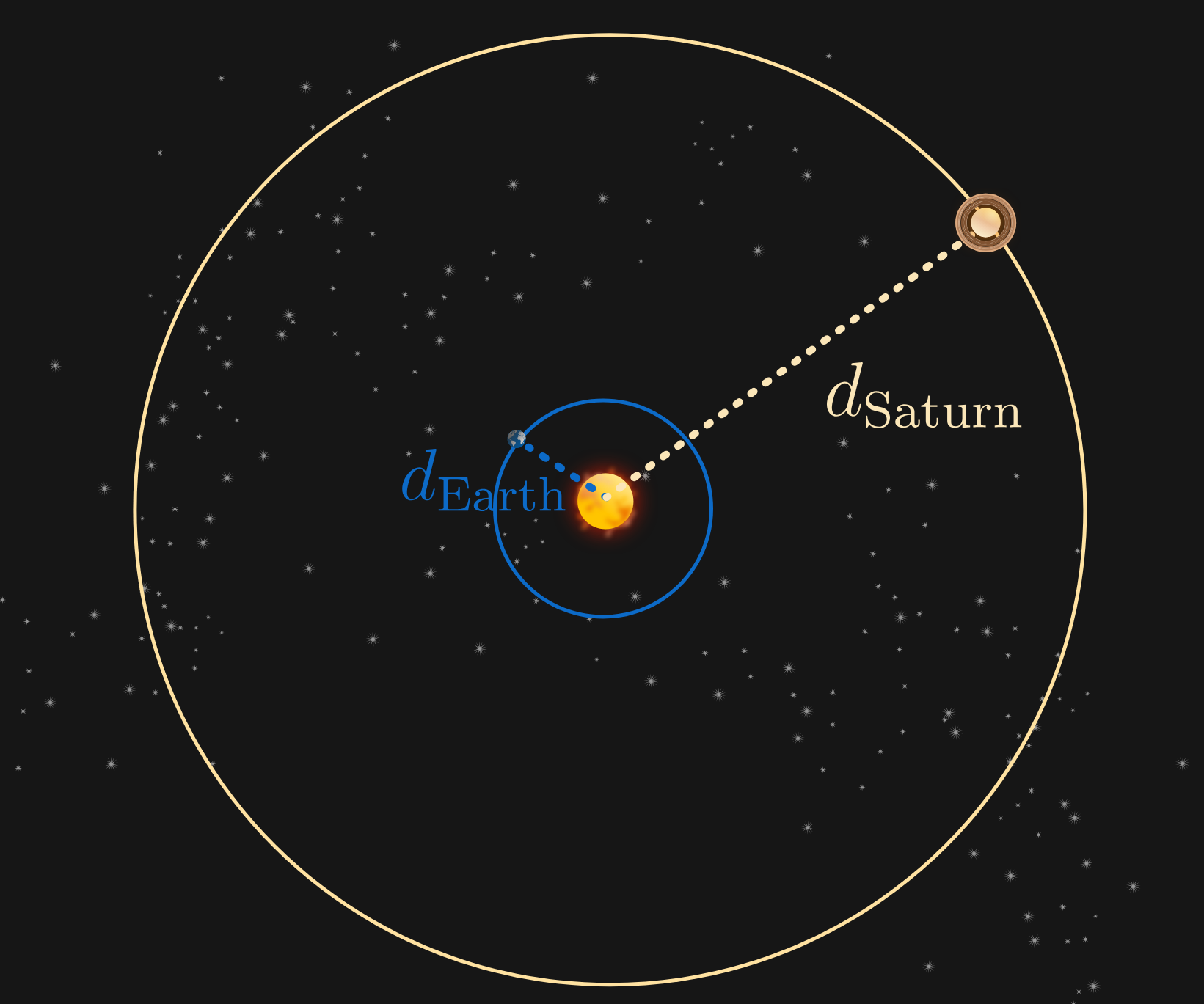Between the Sun and which planet, Earth or Saturn, is the gravitational force larger?


Details and Assumptions:

• Rather than writing out $d_\text{Earth}$ in meters, as in the previous question, astronomers define this distance to be $1$ astronomical unit or $\SI{1}{au}.$ Thus, Saturn's orbital distance from the Sun is $d_\text{Saturn}=\SI{9.57}{au}.$

Gravity

Calculating gravitational forces in our solar system requires knowing $M_\text{Sun},$ so you might wonder how astronomers first measured the Sun's mass. Putting it on a scale just wasn't an option, so they used Newton's theory of gravity, and measurements of Earth's orbital motion. Let's see how this works.

If we assume that Earth's orbit is circular (a pretty good approximation), then its acceleration $a = v^2/d_\text{Earth},$ where $v$ is Earth's speed in its orbit and $d_\text{Earth}$ is its distance from the Sun.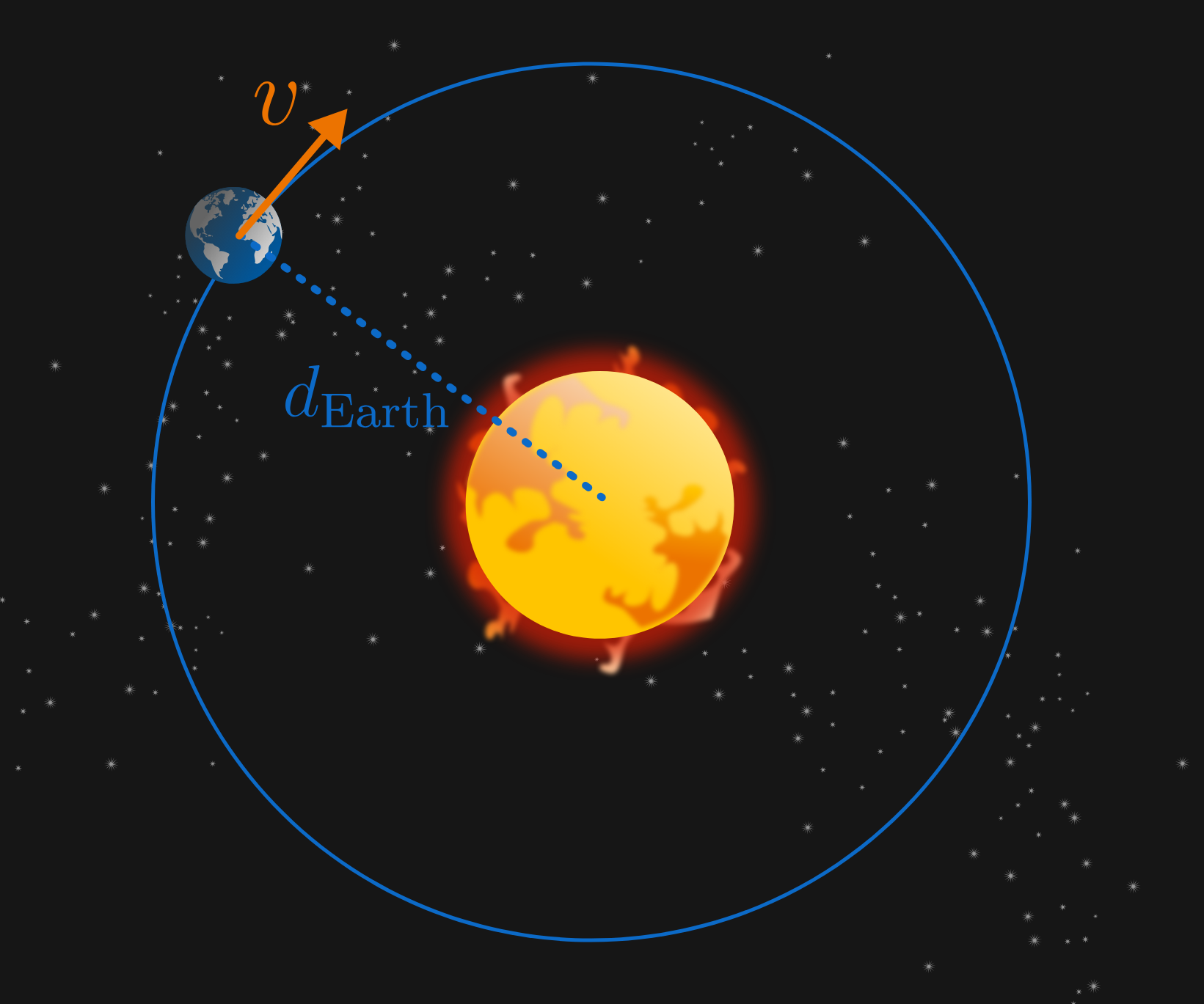Newton's law of motion relates acceleration to the net force on the Earth, which is the gravitational force. It says $F_g=M_\textrm{Earth}a=\frac{M_\textrm{Earth} v^2}{d_\text{Earth}},$ so Newton's law of motion gives us an alternate way to compute the gravitational force on the Earth, without using Newton's gravitational law$^1$.

We first need to estimate Earth's orbital speed $v.$ If Earth orbits the Sun once per $365$ days on a circular orbit with radius $d_\text{Earth}=\SI{1.5e8}{\kilo\meter},$ what is its speed $v$ in $\si[per-mode=symbol]{\kilo\meter\per\second}?$


Details and Hint:

• The distance Earth travels around its orbit is $2\pi d_\text{Earth}.$
• The units of the answer will be $\si[per-mode=symbol]{\kilo\meter\per\second}$ if you first convert $365$ days into $\si{\second}$ and express the distance in $\si{\kilo\meter}.$

$^1$ With his name on several laws, Newton was a busy guy in the 1600's.

Gravity

Now that we have estimated the Earth's orbital velocity, a little algebra will lead us straight to the mass of the Sun.We know that gravity is the force that pulls on Earth as it orbits the Sun, so in Newton's law of motion, $F_g = M_\textrm{Earth}a,$ we can substitute $F_g=GM_\textrm{Earth}M_\textrm{Sun}/d_\textrm{Earth}^2:$

\begin{aligned} F_g &= M_\textrm{Earth} \frac{v^2}{d_\textrm{Earth}} \\ \frac{GM_\textrm{Sun} M_\textrm{Earth}}{d_\textrm{Earth}^2} &= M_\textrm{Earth} \frac{v^2}{d_\textrm{Earth}}. \end{aligned}

Although this relationship may appear complicated, we can solve it for $M_\text{Sun},$ and calculate it, without any more information than we've already used.

Solve the above equation for $M_\textrm{Sun}.$

Gravity

Astronomers routinely use orbital motion to deduce the masses of stars like the Sun, as well as much larger objects like galaxies; however, in this course, we are more interested in gravity's role energizing stars and other celestial objects, rather than the fine details of motion under gravity's influence.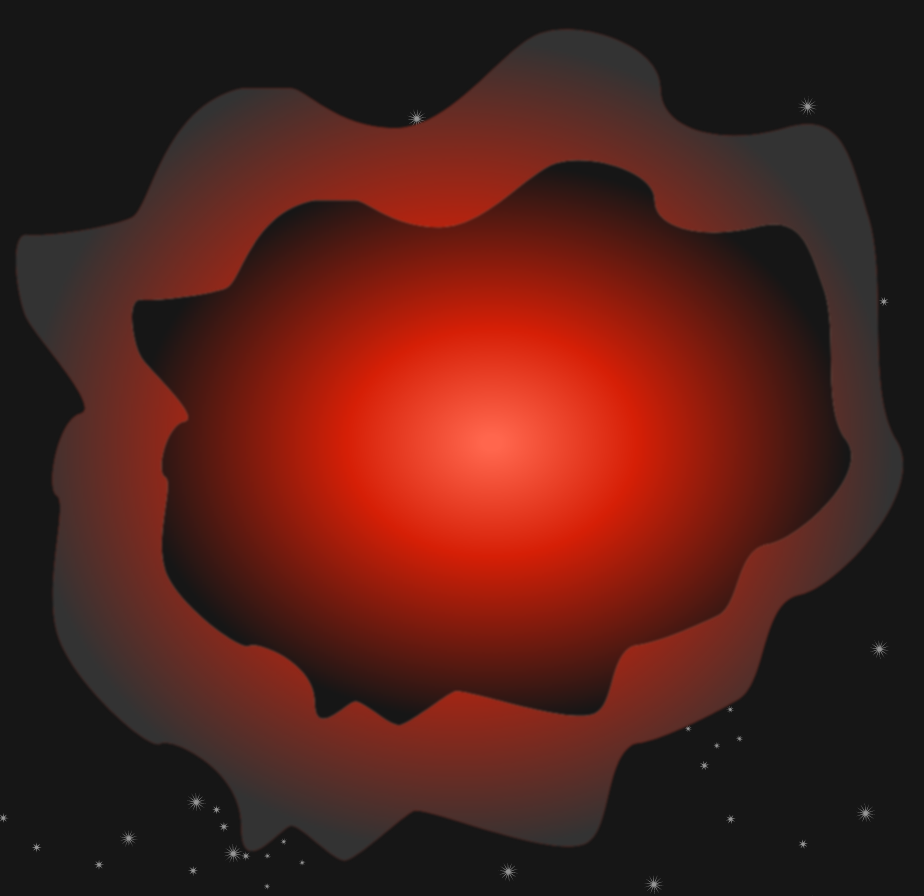In the remainder of this quiz, we will examine the birth of our solar system from a diffuse cloud of gas and debris, leftover from a star whose existence ended much earlier in a cataclysmic supernova explosion. We will work out an important relationship between gravitational potential energy and kinetic energy—sometimes called the virial theorem—that we will employ later in this course when we examine the life cycle of a star.

Gravity

Several billion years ago, all of the mass that makes up the Sun and planets in our solar system was spread out as a low-density gas mixed with dust called a nebula.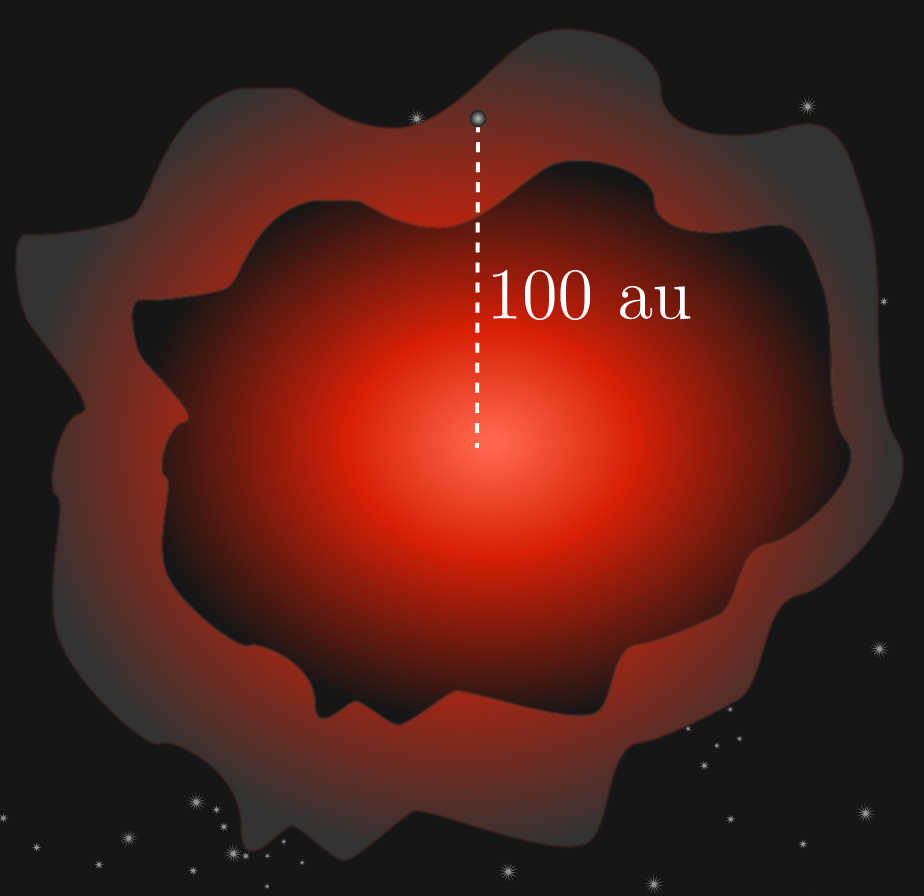Which stage of the solar system has lower gravitational potential energy—the spread-out gas, or our solar system today?


Details and Assumptions:

• The Sun has a radius less than $\SI{1}{au},$ whereas the region of gas that formed our solar system is roughly spherical with radius $\SI{100}{au}.$
• At the edge of the nebula or on the surface of the Sun, a gas molecule feels a gravitational force toward the center of the system. As a force like gravity does work it loses potential energy.

Gravity

Let's consider a $\SI{1}{\kilo\gram}$ lump of metal as it is pulled from the outer fringe of the early solar system to a radius where it ultimately ends up in Earth's crust.

The metal lump is pulled from $\SI{100}{au}$ to $\SI{1}{au}$ as the nebula collapses. How much potential energy $($ in $\text{MJ})$ does it lose, $\lvert \Delta U_g \rvert ?$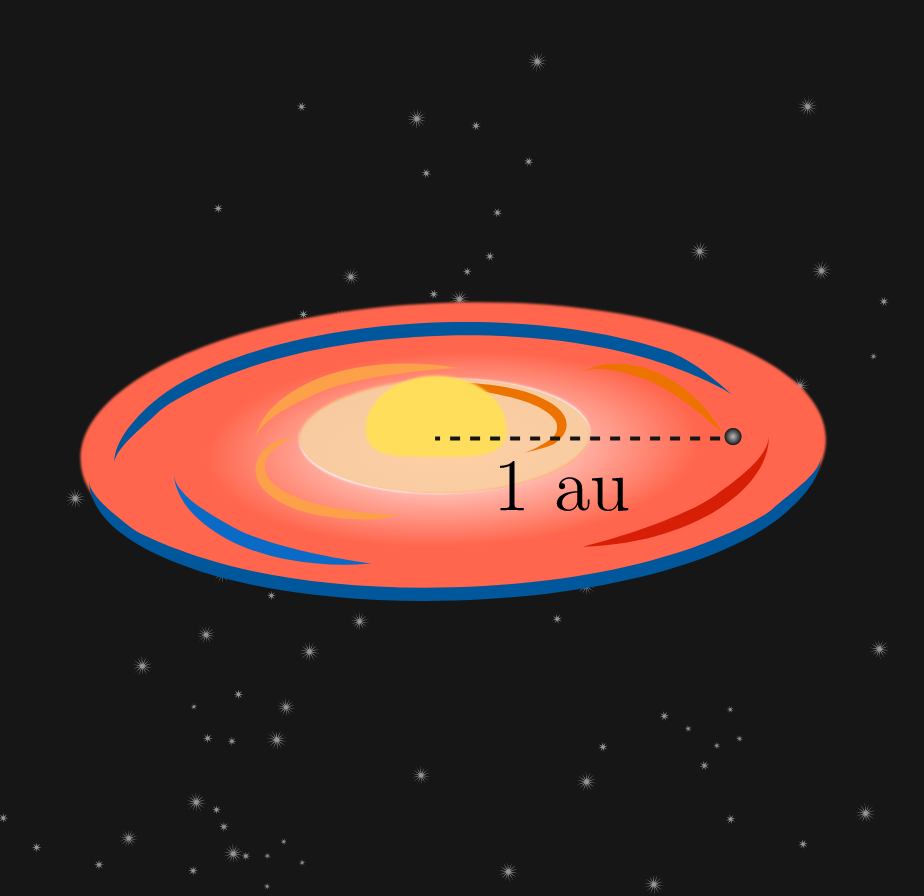Details and Assumptions:

• The gravitational potential energy of the lump with mass $m$ that is a distance of $r$ from the center of another object with mass $M$ is $U_g=-\frac{Gm M}{r},$ where $G=\SI[per-mode=symbol]{6.67e-11}{\meter\cubed\per\kilo\gram\per\second\squared}.$
• Take $M=\SI{1.99e30}{\kilo\gram},$ the mass of the solar system.
• $\SI{1}{au}=\SI{1.5e11}{\meter}.$
• Enter your answer (a positive number) in mega-Joules: $\SI{1}{\mega\joule}=\SI{10^6}{\joule}$.

Gravity

Today, the lump of metal that we've tracked is now moving along with Earth with the kinetic energy

$K=\frac12 M v^2,$

where $M$ is the mass of the lump, and $v$ is the orbital speed of Earth that you calculated earlier in this quiz.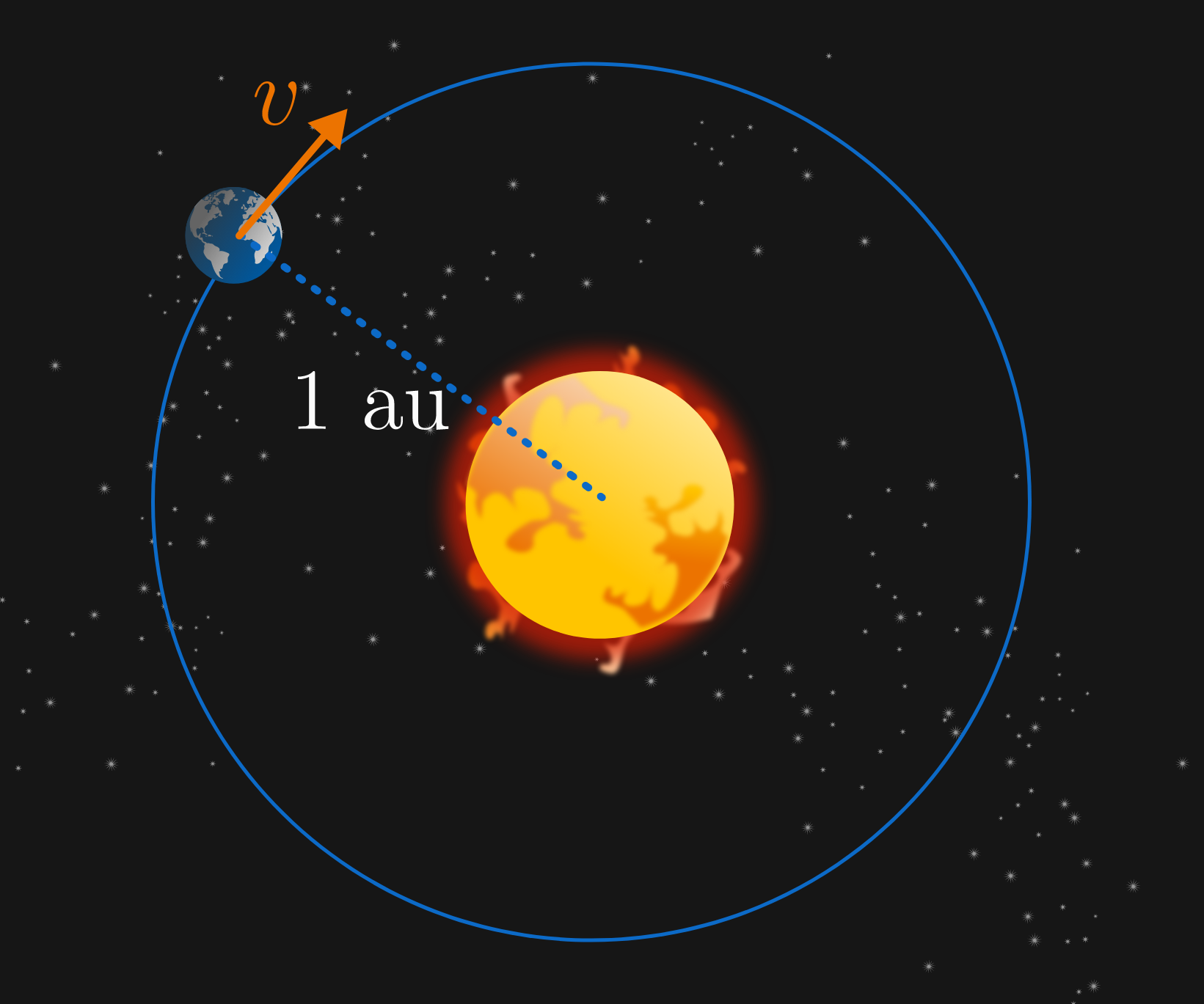If you calculate the kinetic energy, you will find that it is less than $\lvert \Delta U_g\rvert,$ which you calculated in the previous question. Because energy is conserved, we can assume the rest of the energy it lost was dissipated and reabsorbed by Earth as heat:

$\lvert \Delta U_g \rvert = K + \text{Heat}.$

About what percent of the potential energy lost by the metal lump was released as heat?


Details and Assumptions:

• Neglect Earth's spin around its axis.

Gravity

Earth's core, which is made of a mixture of dense molten metals, including iron and nickel, is very hot. Heating initially occurred as bits of dust and gas molecules lost potential energy during gravitational collapse of the cloud. In this quiz, we've calculated just $\SI{1}{\kilo\gram}$ of this material can release hundreds of $\si{\mega\joule}$ of energy.

We also discovered an important result that we will use several times in this Exploration. Specifically, the kinetic energy $K$ of the metal lump was about half of the potential energy $\lvert U_g \rvert$ it lost. In fact, if we had been more careful about including the lump's initial kinetic energy, we would have found that exactly half of the potential energy was lost as heat. This statement is formally called the virial theorem. We will see in a later chapter that stars always run the risk of gravitational collapse, and the virial theorem provides us a way to understand what happens to a star's temperature during collapse.

Gravity

×